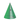# Cone Volume and Area Calculator

Height

Select Unit### Calculate Cone Volume ?

The Volume of a Cone is:Volume = π * radius2 * height/3 .
ex ; Volume = π * 102 * 20/3 = 2094.395 m3

### Slant height of a Cone ?

Slant Cone = (radius2 + height2)
ex : Slant Height = √102+202= 22.37m

### Cone area ?

The Lateral Area of a Cone is :lateral Surface = π * radius * √(height2+radius2).
ex : Lateral area = π * 10 √202+102= 702.48 m2

The Surface Area of a Cone is :Surface area = π * radius * slant + π * radius2

### Base Area of Cone ?

Base area of a Cone is :Base area = π * radius2
ex: Base area = π * 102 = 314.16m2